# Math formulas

Here you will learn about math formulas, including what math formulas are and how to use them.

Students will first learn about math formulas as part of measurement and data in 4th grade and 5th grade, and expand on that knowledge in geometry in 6th, 7th, and 8th grade.

## What are math formulas?

Math formulas are rules that connect two or more variables. They are used to work out the values of unknown variables by substituting in other known values.

For example, here is a rectangle with base b and height h.

The formula for the perimeter P of a rectangle is:

P=2 \, (b+h)

We can use this formula to find the value of the perimeter by substituting in the values for the length and width of the rectangle.

### List of math formulas

These are some of the measurement and geometry formulas you are most likely to encounter in grades 4-8.

Area \hspace{1.9cm}

Area of a rectangle

A=b \times h

Where A is area, b is the base
and h is the height.

Area of a square

A=s \times s

Where A is area and s is side.

Area of a triangle

A=\cfrac{1}{2} \, b h

Where A is area, b is base and
h is height.

Area of a parallelogram

A=b \times h

Where A is area, b is base and
h is height.

Area of a circle

A=\pi \times r^2

Where A is area and r is radius.

Area of a trapezoid

A=\cfrac{1}{2} \, \left(b_1+b_2\right) h

Where A is area, b_1 is base 1,
b_2 is base 2 and h is height.

Circumference \hspace{1.9cm}

Circumference of a circle

C=\pi d \;\;
C=2 \pi r

Where C is circumference,
d is diameter, r is radius.

Perimeter \hspace{1.9cm}

Perimeter of a rectangle

P=2(b+h)

Where P is perimeter, b is the
base and h is the height.

Perimeter of a square

P=4 s \hspace{1.35cm}
P=s+s+s+s

Where P is perimeter and s is
a side.

Pythagorean Theorem \hspace{1.8cm}

Pythagorean Theorem

c^2=a^2+b^2

Where a is a side, b is a side
and c is the hypotenuse.

Surface Area \hspace{1.9cm}

Surface area of a cube

A=6 s^2

Where A is area, the variable s
is the side and the 6 represents
the number of faces of the cube.

Volume \hspace{1.9cm}

Volume of a
rectangular prism

V=b \times h \times d

Where V is the volume, b is the
base, h is the height and
d is the depth.

Volume of a cube

v=s \times s \times s

Where V is volume and s is
side.

Volume of a cylinder

V=\pi r^2 h

Where V is volume, r is radius
of the circle and h is the height.

Volume of a cone

V=\cfrac{1}{3} \, \pi r^2 h

Where V is volume, r is radius
of the circle and h is the height.

Volume of a sphere

V=\cfrac{4}{3} \, \pi r^3

Where V is volume, and r is

### What are math formulas?## Common Core State Standards

• Grade 4: Measurement and Data (4.MD.A.3)
Apply the area and perimeter formulas for rectangles in real world and mathematical problems.

• Grade 5: Measurement and Data (5.MD.C.5b)
Apply the formulas V = l \times w \times h and V = b \times h for rectangular prisms to find volumes of right rectangular prisms with whole number edge lengths in the context of solving real world and mathematical problems.

Find the volume of a right rectangular prism with fractional edge lengths by packing it with unit cubes of the appropriate unit fraction edge lengths, and show that the volume is the same as would be found by multiplying the edge lengths of the prism.

Apply the formulas V = l \times w \times h and V = b \times h to find volumes of right rectangular prisms with fractional edge lengths in the context of solving real-world and mathematical problems.

Know the formulas for the area and circumference of a circle and use them to solve problems; give an informal derivation of the relationship between the circumference and area of a circle.

Apply the Pythagorean Theorem to determine unknown side lengths in right triangles in real-world and mathematical problems in two and three dimensions.

Know the formulas for the volumes of cones, cylinders, and spheres and use them to solve real-world and mathematical problems.

## How to use math formulas

In order to solve with math formulas:

1. Use the formula given in the question.
2. Work carefully to answer the question, one step at a time.
3. Write the final answer clearly.

## Math formulas examples

### Example 1: perimeter of a rectangle

Find the perimeter of the given rectangle using the formula, P=2 \, (b+h).

1. Use the formula given in the question.

You will use the formula P=2 \, (b+h) to find the perimeter of the rectangle.

The length of the rectangle is 13 \, cm and the width is 5 \, cm, so substitute the measurements into the formula.

P=2 \, (13+5)

2Work carefully to answer the question, one step at a time.

P=2 \, (13+5)

Solve the operation within the parenthesis first. Adding 13 and 5 gives you 18.

P=2 \times 18

You will then multiply 2 \times 18.

This can also be solved as 18 + 18 to give the answer of 36.

P=36

The final answer is P = 36 \, cm.

The perimeter of the rectangle is 36 centimeters.

### Example 2: area of a rectangle

Find the area of the rectangle given, using the formula A = b \times h.

Use the formula given in the question.

Work carefully to answer the question, one step at a time.

### Example 3: area of a square

Find the area of the square given, using the formula A=s \times s.

Use the formula given in the question.

Work carefully to answer the question, one step at a time.

### Example 4: volume of a rectangular prism

Find the volume of the rectangular prism using the formula, V = b \times h \times d.

Use the formula given in the question.

Work carefully to answer the question, one step at a time.

### Example 5: area of a triangle

Find the area of the triangle given, using the formula A=\cfrac{1}{2} \, b h.

Use the formula given in the question.

Work carefully to answer the question, one step at a time.

### Example 6: area of a parallelogram

Find the area of the parallelogram given, using the formula A = b \times h.

Use the formula given in the question.

Work carefully to answer the question, one step at a time.

### Teaching tips for math formulas

• Formulas can be difficult for students to memorize. Always have a way for students to refer back to the formula, such as an anchor chart or a reference sheet, until they get familiar and comfortable with each formula.

• These basic math formulas will prepare students for work in high school with more complex numbers and formulas, including linear equations, the quadratic formula, and other algebra formulas, such as the slope-intercept formula.

• While using quizzes to assess a student’s knowledge of the different equations is important, consider making the assessments real-life, and have students measure items within the classroom or school, and use the formulas to calculate the area of the classroom or playground.

### Easy mistakes to make

• Substituting in the wrong number in the equation
Learning the equation is important, but learning what each variable in an equation is equally important. In some basic math formulas, flipping the base and the height would still give students the same answer. However, as students move to more complex formulas, flipping of variables could result in incorrect answers.

For example, when finding the area of a trapezoid, flipping of a side versus the height would result in the wrong answer because the sides are added together and divided by 2, and the height is not.

• Take care with writing variables clearly
Neat writing is important. For example, the variables v and u can easily be muddled as they look similar and they both represent velocity in kinematic formulas.

### Practice math formulas questions

1. Find the perimeter of the rectangle using the appropriate formula.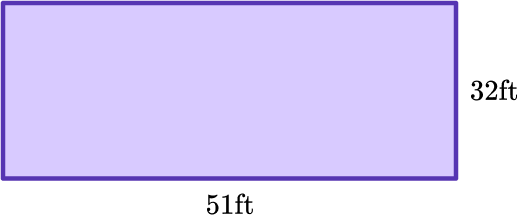83 feet166 feet163 feet85 feetYou will use the formula P=2 \, (b+h) to find the perimeter.

Substitute the values within the formula.

P=2 \, (32+51)

Add the values within the parenthesis first.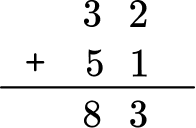P=2 \, (83)

Then multiply 83 by 2.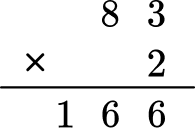The final answer is P = 166 \, ft.

The perimeter of the rectangle is 166 feet.

2. Find the area of the rectangle using the appropriate formula.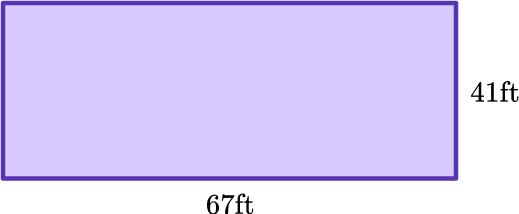2,747 feet^2216 feet^22,647 feet^2225 feet^2You will use the formula A = b \times h to find the area of the rectangle.

Substitute in the values within the formula.

A=41 \times 67

Multiply 41 by 67.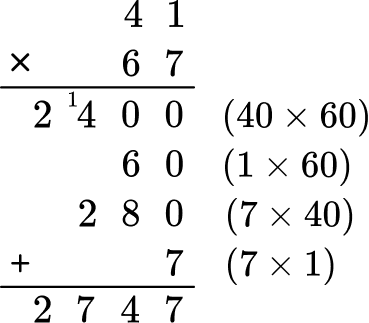The final answer is A = 2,747 \, ft^2 .

The area of the rectangle is 2,747 feet^2.

3. Find the area of the square given, using the appropriate formula.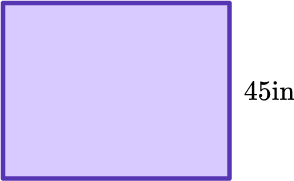180 inches^21,825 inches^22,025 inches^2225 inches^2You will use the formula for finding the area of a square A=s \times s.

Substitute the value into the formula.

A=45 \times 45

You will multiply 45 by 45.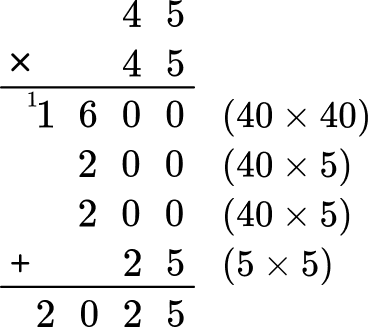The final answer is A = 2,025 \, in^2 .

The area of the square is 2,025 inches^2.

4. Find the volume of the rectangular prism using the appropriate formula.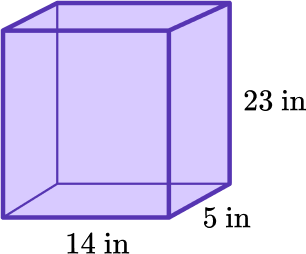322 inches^31,510 inches^3525 inches^31,610 inches^3You will use the formula to find volume of the rectangular prism V=l \times w \times h.

Substitute the measurements into the given formula.

V=14 \times 5 \times 23

Multiply 14 \times 5, first.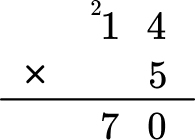Then multiply the product of 14 and 5 by 23.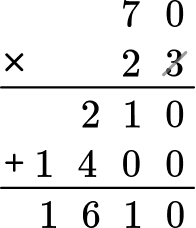The final answer is V= 1,610 \, in^3 .

The volume of the rectangular prism is 1,610 inches^3.

5. Find the area of the triangle using the appropriate formula.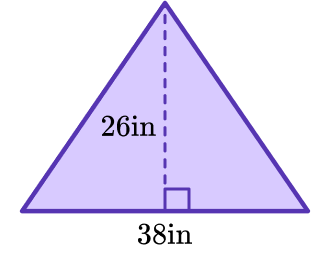988 inches^2114 inches^2494 inches^2524 inches^2You will use the formula A=\cfrac{1}{2} \, b h to find the area of the triangle.

Substitute the measurements into the formula.

A=\cfrac{1}{2} \, (38 \times 26)

Following the order of operations, multiply 38 by 26 first.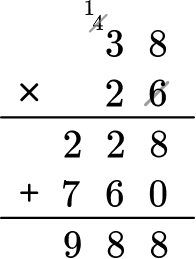A=\cfrac{1}{2} \, (988)

Then multiply 988 by \,\cfrac{1}{2} \, , or divide 988 by 2.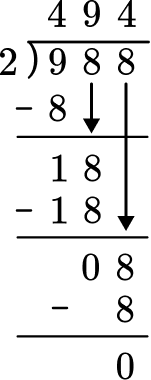The final answer is A= 494 \, in^2 .

The area of the triangle is 494 inches^2.

6. Find the area of the parallelogram using the appropriate formula.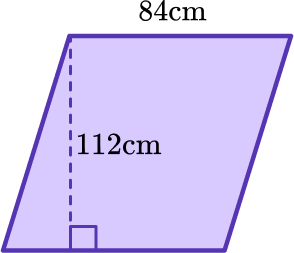9,408 \, cm^2.448 \, cm^2.623 \, cm^2.9,308 \, cm^2.You will use the formula A=b \times h to find the area of the triangle.

Substitute the measurements into the formula.

A=84 \times 112

You will multiply 112 and 84 together to find the area of the quadrilateral.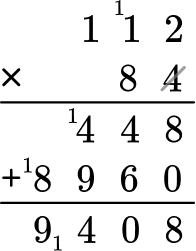The final answer is A= 9,408 \, cm^2 .

The area of the parallelogram is 9,408 centimeters ^2.

## Math formulas FAQs

What is the Pythagorean Theorem?

The Pythagorean Theorem states that in any right triangle, the sum of the squares of the legs equals the square of the hypotenuse. If you know the lengths of any two sides of a right triangle, you can use the Pythagorean Theorem to find the third length.

What are the six functions of trigonometry?

The six functions of an angle, used in trigonometry, are sine, cosine, tangent, cotangent, secant, and cosecant.

What is the distance formula?

The distance formula is a formula used to measure how far apart two objects or points are.

## Beyond elementary

You can use formulas in lots of different areas of math. One of the most famous formulas is the quadratic formula which we can use to solve quadratic equations.

x=\cfrac{-b \pm \sqrt{b^2-4 a c}}{2 a}

Quadratics are a type of polynomial and using coordinate geometry you can use them to produce a parabola shaped quadratic graph. We can use the graph to work out the solutions to the equation and the y-intercept of the graph.

## Still stuck?

At Third Space Learning, we specialize in helping teachers and school leaders to provide personalized math support for more of their students through high-quality, online one-on-one math tutoring delivered by subject experts.

Each week, our tutors support thousands of students who are at risk of not meeting their grade-level expectations, and help accelerate their progress and boost their confidence.